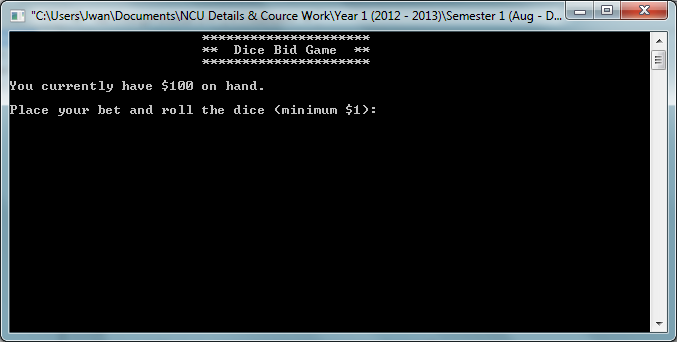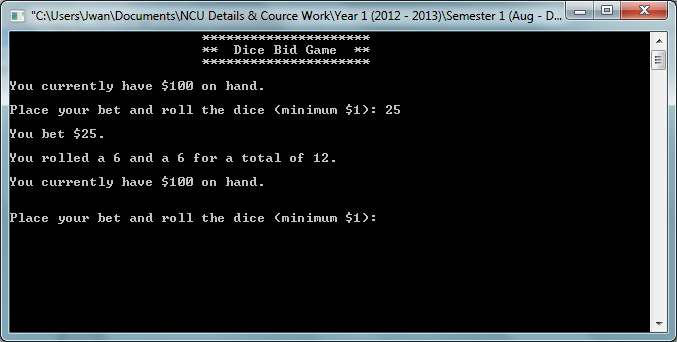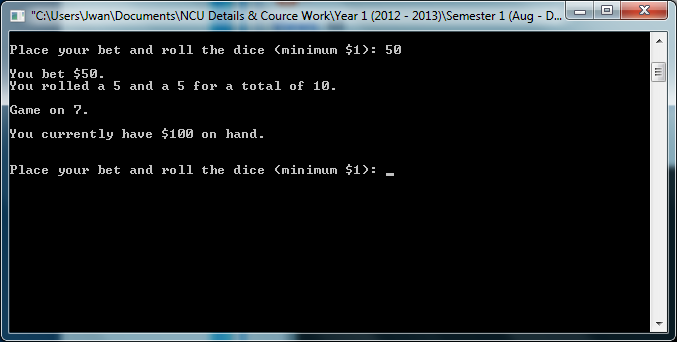Dice Bid GamePosted by JanWan Last Updated: September 19, 20122227

# include <iostream>
# include <cmath>
# include <iomanip>
# include <cstdlib>

using namespace std;

int main()
{
int die1;
int die2;
int dice;
int bet;
int point;
int money = 100;
char roll;

cout<<"\t\t\t*********************"<<endl;
cout<<"\t\t\t**  Dice Bid Game  **"<<endl;
cout<<"\t\t\t*********************"<<endl<<endl;

do
{
cout << "You currently have \$" << money << " on hand.";
cout<<endl;

cout << "\nPlace your bet and roll the dice (minimum \$1): ";
cin >> bet;
cout<<endl;

while (bet < 1 || bet > money)
{
if (bet < 1)
cout << "\nDont be so cheap put some money on the table!";
if (bet > money)
cout << "\nYou don't have that much!";
cout<<endl;
cout << "\n\nPlace your bet and roll the dice (minimum \$1): ";
cin >> bet;
cout<<endl;
}

cout << "You bet \$" << bet << ".";
cout<<endl;

cin.get(roll);

die1 = rand() % 6 + 1;
die2 = rand() % 6 + 1;
dice = die1 + die2;

cout << "\nYou rolled a " << die1 << " and a " << die2
<< " for a total of " << dice << "."<<endl;
cout<<endl;

if (dice == 7 )
{
cout << "Youve won";
cout<<endl;
money += bet;
}

if (dice == 11)
{
cout << "Youve lost";
cout<<endl;
money -= bet;
}

else
{
do
{
cout << "You currently have \$" << money << " on hand."<<endl; //copied the betting loop you had
cout<<endl;
cout << "\nPlace your bet and roll the dice (minimum \$1): ";
cin >> bet;
cout<<endl;

while (bet < 1 || bet > money)
{
if (bet < 1)
cout << "\nC'mon, take a chance!";
if (bet > money)
cout << "\nYou don't have that much!";
cout << "\n\nPlace your bet (minimum \$1): ";
cin >> bet;
}
cout << "You bet \$" << bet << "."; //end betting loop

cin.get(roll);

die1 = rand() % 6 + 1;
die2 = rand() % 6 + 1;
dice = die1 + die2;

cout << "\nYou rolled a " << die1
<< " and a " << die2
<< " for a total of " << dice << ".";
cout<<endl;

if (dice == 7)
{
cout << "\nYOU WON!!!! lets raise the Stakes"<<endl;
money += bet;
}

if (dice == 11)
{
cout << "\nYOU LOSE its ok you can WIN it back"<<endl;
money -= bet;
}

else
{
cout << "\nGame on 7. "<<endl;
cout<<endl;

}

} while (dice != point || dice != 7);
}
} while (money > 0);

cout << "/nLooks like you ran out of money. "
<< "Thanks for playing!" << endl;

system("pause");
}

J.W. Productioncrazy dice code, it's a random variable selection gamenice code broDisplay screen for dice game Place your bet by entering the amount you want to bet out of the \$100.A bet of \$25 was madeAnother bet of \$50 was madeThese screen shot are for the source code above.